# Chapter 11 - Distillation¶

## Page 548 Example 11.1¶

In :
p0_A=106#  #Vapour pressure of n-heptane  in kN/m**2
p0_B=73.7#  #Vapour pressure of toluene  in kN/m**2
P=101.3#  #Total pressure in kN/m**2

xA=(P-p0_B)/(p0_A-p0_B)

yA=p0_A*xA/P

print"\nMole fraction in liquid phase is : %.3f"%(xA)
print"\nMole fraction in vapour phase is : %.3f\n"%(yA)

Mole fraction in liquid phase is : 0.854

Mole fraction in vapour phase is : 0.894



## Page 549 Example 11.2¶

In :
from math import log,exp

Pc=4700#  #Critical pressure in kN/m**2
Tc=508.1#  #critical temperature in K
p1=100.666#  #in kN/m**2
T1=329.026#  #in K
T=350.874#  #in K

Tr1=T1/Tc
Pr1=p1/Pc
print"\nTr1 = %.3f   \nPr1 = %.3f  \n"%(Tr1,Pr1)

c5=-35+(36/Tr1)+(42*log(Tr1))-(Tr1**6)
c2=((0.315*c5)-log(Pr1))/((0.0838*c5)-log(Tr1))
c1=0.0838*(3.758-c2)
print"\nc5 = %.4f \nc2 = %.4f \nc1 = %.4f\n"%(c5,c2,c1)

k9=-35*c1
k10=-36*c1
k11=(42*c1)+c2
k12=-c1

print"\nk9 = %.3f \nk10 = %.3f \nk11 = %.4f"%(k9,k10,k11)
print"\nk12 = %.5f\n"%(k12)

Tr=T/Tc
Pr=exp(k9-(k10/Tr)+(k11*log(Tr))+(k12*Tr**6))
p0=Pc*Pr
print"\nPr = %.3f   \n\nP0 = %.2f kN/m**2\n"%(Pr,p0)

Tr1 = 0.648
Pr1 = 0.021

c5 = 2.2687
c2 = 7.2970
c1 = -0.2966

k9 = 10.380
k10 = 10.677
k11 = -5.1589

k12 = 0.29657

Pr = 0.043

P0 = 203.75 kN/m**2



## Page 549 Exaple 11.3¶

In :
k1_B=6.90565
k2_B=1211.033
k3_B=220.79

k1_T=6.95334
k2_T=1343.943
k3_T=219.377

t=338-273#  #in Degree celsius
P=101.3#  #in kN/m**2
xB=0.5
xT=0.5

def antoine(k1,k2,k3,T):
p0=10**(k1-(k2/(T+k3)))
return p0

p0_B=antoine(k1_B,k2_B,k3_B,t)*101.325/760
p0_T=antoine(k1_T,k2_T,k3_T,t)*101.325/760

print"\nP0_B = %.1f kN/m**2\nP0_T = %.1f kN/m**2\n"%(p0_B,p0_T)

pB=xB*p0_B
pT=xT*p0_T
print"\npB = %.2f kN/m**2 \npT = %.3f\n"%(pB,pT)

p=pB+pT
yB=pB/p
yT=pT/p
print"\nyB = %.3f \nyT = %.3f\n"%(yB,yT)

P0_B = 62.1 kN/m**2
P0_T = 22.5 kN/m**2

pB = 31.05 kN/m**2
pT = 11.254

yB = 0.734
yT = 0.266



## Page 550 Example 11.4¶

In :
def antoine(k1,k2,k3,T):
p0=10**(k1-(k2/(T+k3-273)))
return p0

k1_B=6.90565
k2_B=1211.033
k3_B=220.79

k1_T=6.95334
k2_T=1343.943
k3_T=219.377

xB=0.5
xT=0.5

print"\n\tT(K)"
T=[373, 353, 363, 365, 365.1]
for t in T:
print t,'\t',

print
i=0

p0_B=[];p0_T=[];pB=[];pT=[];p=[]
while i<5:
p0_B.append(antoine(k1_B,k2_B,k3_B,T[(i)])*101.325/760)
p0_T.append(antoine(k1_T,k2_T,k3_T,T[(i)])*101.325/760)
pB.append(xB*p0_B[(i)])
pT.append(xT*p0_T[(i)])
p.append(pB[(i)]+pT[(i)])
i=i+1

print"\n\t\np0 B:"
for pp in p0_B:
print "%0.3f\t"%pp,
print"\n\t\np0 T:"
print
for pp in p0_T:
print "%0.3f\t"%pp,
print
print"\n\t\npB:"
print
for pp in pB:
print "%0.3f\t"%pp,

print"\n\t\npT:"
print
for pp in pT:
print "%0.3f\t"%pp,

print"\n\t\npB+pT:"
print
for pp in p:
print "%0.3f\t"%pp,

print
#since total pressure at 365.1 K is nearly same as 101.3 kPa
print"\nBoiling temperature is %.3f   K"%(T)

	T(K)
373 	353 	363 	365 	365.1

p0 B:
180.051	101.013	136.121	144.159	144.570

p0 T:

74.170	38.825	54.227	57.825	58.010

pB:

90.025	50.507	68.060	72.079	72.285

pT:

37.085	19.412	27.113	28.912	29.005

pB+pT:

127.110	69.919	95.174	100.992	101.290

Boiling temperature is 365.100   K


## Page 551 Example 11.5¶

In :
def antoine(k1,k2,k3,T):
p0=10**(k1-(k2/(T+k3-273)))
return p0

k1_B=6.90565
k2_B=1211.033
k3_B=220.79

k1_T=6.95334
k2_T=1343.943
k3_T=219.377

#Since total pressure is 101.3 kPa, pB=pT
pB=50.65
pT=50.65

print"\nT(K) : "
T=[373.2 ,371.2 ,371.7 ,371.9, 372]
for t in T:print t,'\t',

i=0

p0_B=[];p0_T=[];xB=[];xT=[];x=[]
while i<5:
p0_B.append(antoine(k1_B,k2_B,k3_B,T[(i)])*101.325/760)
p0_T.append(antoine(k1_T,k2_T,k3_T,T[(i)])*101.325/760)
xB.append(pB/p0_B[(i)])
xT.append(pT/p0_T[(i)])
x.append(xB[(i)]+xT[(i)])
i=i+1

print"\np0 B:"
for p in p0_B: print p,'\t',
print
print"\np0 T:"
for p in p0_T:print p,'\t',
print
print"\nxB:"
for xx in xB:print xx,'\t',
print
print"\nxT:"
for xx in xT:print xx,'\t',
print
print"\nx = xB+xT:"
for xx in x:print xx,'\t',
print
#Since last value is closer to 1, 372 K is the required dew point
print"\nDew point can be taken as %.3f   K"%(T)

T(K) :
373.2 	371.2 	371.7 	371.9 	372
p0 B:
181.028525362 	171.432167447 	173.793590263 	174.745148387 	175.222430638

p0 T:
74.6211877248 	70.2066082135 	71.2902696145 	71.7274367157 	71.9468173127

xB:
0.279790159583 	0.2954521357 	0.291437675713 	0.289850679504 	0.289061165375

xT:
0.678761643232 	0.721442059214 	0.71047564098 	0.706145407102 	0.703992224977

x = xB+xT:
0.958551802815 	1.01689419491 	1.00191331669 	0.995996086606 	0.993053390352

Dew point can be taken as 372.000   K


## Page 557 Example 11.6¶

In :
#Fractional vapourisation   f=V/F
f=0.25

slope=(1-f)/-f

#from fig 11.9, a construction is made
print"\nFor slope = %d"%(slope)
print"\nx=0.42 \t y=0.63\n"

#from fig. 11.10,temperature corresponding to x=0.42 is noted
print"\nfor x=0.42 \t T=366.5"

For slope = -3

x=0.42 	 y=0.63

for x=0.42 	 T=366.5


## Page 564 Example 11.7¶

In :
from scipy.optimize import fsolve
%matplotlib inline
from matplotlib.pyplot import plot,title,xlabel,ylabel,show
from numpy import arange

# Find Number of theoretical plates needed and the position of entry for the feed

F = 100#                #Feed [kmol]

def Feed(x):
f=[]
f.append(x+x-100)                    #Overall mass Balance
f.append(0.9*x+.1*x-(100*.4))#        #A balance on MVC,benzene
return f
x = [50, 50]
product = fsolve(Feed,x)

#Using notation of figure 11.13
Ln = 3*product
Vn = Ln + product

#Reflux to the plate
Lm = Ln + F
Vm = Lm - product

#Equilibrium Composition
xt = .79
yt = .9
#From Top eqm line
yt1 = (Ln/Vn)*xt + (product/Vn)
xt1=.644#        #Thus from Eqm curve for yt1
#From Top eqm line
yt2 = (Ln/Vn)*xt1 + (product/Vn)
xt2=.492#        #Thus from Eqm curve for yt2
#From Top eqm line
yt3 = (Ln/Vn)*xt2 + (product/Vn)
xt3=.382#        #Thus from Eqm curve for yt3
#From II Eqm Line
yt4 = (Lm/Vm)*xt3 - (product/Vm)*.1
xt4=.2982#        #Thus from Eqm curve for yt4
#From II Eqm Line
yt5 = (Lm/Vm)*xt4 - (product/Vm)*.1
xt5=.208#        #Thus from Eqm curve for yt5
#From II Eqm Line
yt6 = (Lm/Vm)*xt5 - (product/Vm)*.1
xt6=.120#        #Thus from Eqm curve for yt6
#From II Eqm Line
yt7 = (Lm/Vm)*xt6 - (product/Vm)*.1
xt7=.048#        #Thus from Eqm curve for yt7

#Equilibrium Data
y=[0, yt7 ,yt6 ,yt5 ,yt4 ,yt3 ,yt2 ,yt1 ,yt]
x=[0, xt7 ,xt6 ,xt5 ,xt4 ,xt3 ,xt2 ,xt1, xt]
#Top Equilibrium Line equation 11.35
x1 = arange(0,.79,100)
y1 = (Ln/Vn)*x1 + (product/Vn)
#Equilibrium Line equation 11.37
x2 = arange(0.048,.44,100)
y2 = (Lm/Vm)*x2 - (product/Vm)*.1

plot(x,y,x1,y1,x2,y2)
title("Lewis-Sorel Method")
xlabel( "Mole fraction of C6H6 in Liquid (x)")
ylabel( "Mole Fraction C6H6 in Vapor (y)")
show()
print"\n\n As the least point on equilibrium Line xt-7 correspond to reboiler, and there will be seven plates"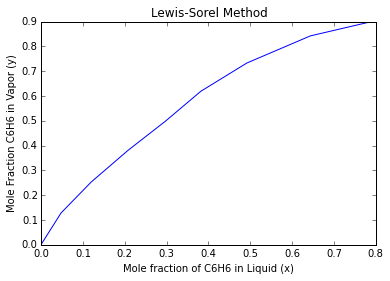As the least point on equilibrium Line xt-7 correspond to reboiler, and there will be seven plates


## Page 567 Example 11.8¶

In :
from scipy.optimize import fsolve
%matplotlib inline
from matplotlib.pyplot import plot,title,xlabel,ylabel,show
from numpy import arange

# Find Number of theoretical plates needed and the position of entry for the feed by mccabe thiele method

F = 100#                #Feed [kmol]

def Feed(x):
f=[]
f.append(x+x-100)#                    #Overall mass Balance
f.append(0.9*x+.1*x-(100*.4))        #A balance on MVC,benzene
return f
x = [50, 50]
product = fsolve(Feed,x)

#Using notation of figure 11.13
Ln = 3*product
Vn = Ln + product

#Reflux to the plate
Lm = Ln + F
Vm = Lm - product

#Equilibrium Data
y=[0, .127, .252 ,.379 ,.498, .594, .708, .818, .9, 1]
x=[0 ,.048, .12, .208, .298, .382 ,.492, .644, .79, 1]
#Diagnol Line
y3 = [0, 1]
x3 = [0 ,1]
#Top Equilibrium Line equation 11.35
x1 = arange(0,.985,100)
y1 = (Ln/Vn)*x1 + (product/Vn)
#Equilibrium Line equation 11.37
x2 = arange(0.048,.44,100)
y2 = (Lm/Vm)*x2 - (product/Vm)*.1

#Setting initial point A x =  .985 at top eqm line
xm = [.985, .965, .965, .92 ,.92 ,.825 ,.825 ,.655 ,.655 ,.44 ,.44 ,.255 ,.255 ,.125 ,.125 ,.048]
ym = [.985 ,.985, .965, .965, .92 ,.92 ,.825 ,.825 ,.655 ,.655 ,.44 ,.44 ,.255, .255, .125, .125]
xp = [.985 ,.965 ,.92 ,.825, .655, .44 ,.255 ,.125 ,.048]
yp = [.985, .965 ,.92 ,.825 ,.655 ,.44, .255, .125, .048]
plot(x,y,x3,y3,x1,y1,x2,y2,xm,ym)
title("Mccabe Thiele Method")
xlabel( "Mole fraction of C6H6 in Liwuid (x)")
ylabel( "Mole Fraction C6H6 in Vapor (y)")
show()
#for i in range(1:8):
plot(xp[1:8],yp[1:8])
title("Equilibrium plot")
xlabel("mole fraction C6H6 in liquid(x)")
ylabel("mole fractionC6H6 in vapour(y)")
show()

print"\n\n The Number of stages are then counted highlighted points that is number of plates required as 7"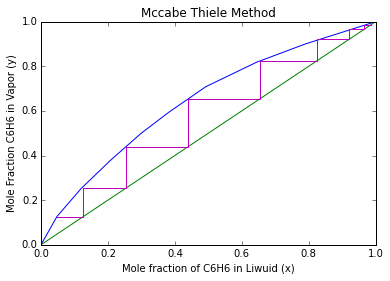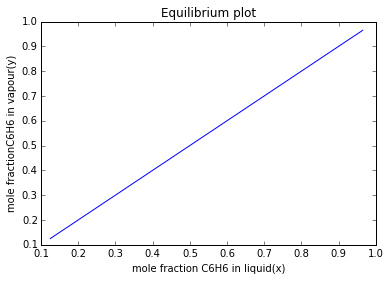The Number of stages are then counted highlighted points that is number of plates required as 7


## Page 574 Example 11.9¶

In :
from math import log

xA_d=0.9
xA_s=0.1
xB_d=1-xA_d
xB_s=1-xA_s

a=2.4
xd=0.9
xf=0.4
xw=0.1

n=(log(xA_d*xB_s/(xB_d*xA_s))/log(a))-1
print"\nNo. of theoretical plates in column is : %d"%(n)

Rm=((xd/xf)-(a*((1-xd)/(1-xf))))/(a-1)
print"\nMinimum reflux ratio is %.2f\n"%(Rm)

yf=0.61
print"\nUsing Graphical construction with yf=0.61\n"
Rmin=(xd-yf)/(yf-xf)
print"Minimum reflux ratio is %.2f\n"%(Rmin)

No. of theoretical plates in column is : 4

Minimum reflux ratio is 1.32

Using Graphical construction with yf=0.61

Minimum reflux ratio is 1.38



## Page 587 Example 11.10¶

In :
from __future__ import division
#From material balance
#  D+W=1
#  0.995D+0.1W=1*3

A=[[1, 1],[0.995, 0.1]]
B=[,]
Rm = (1952-1547)/(1547-295)
print"\n Rm = %.3f"%(Rm)
NA = 1.08*405
print"\n Since the actual reflux is 8 pre cent above the minimum NA = 1.08*NmA = %.3f"%(NA)
N = 5/0.6
print"\n Number of plates to be  required are %.3f"%(5/0.6)

Qb_W = 582 - (-209)
print"\n Heat input to the boiler per unit mass of bottom product is %.3f"%(Qb_W)
print"\n Heat input to the boiler = %.3f kW"%(791*0.78)
print"\n Condenser duty = %d kW"%((1984-296)*0.22)

 Rm = 0.323

Since the actual reflux is 8 pre cent above the minimum NA = 1.08*NmA = 437.400

Number of plates to be  required are 8.333

Heat input to the boiler per unit mass of bottom product is 791.000

Heat input to the boiler = 616.980 kW

Condenser duty = 371 kW


## Page 590 Example 11.11¶

In :
from numpy import mat

#    F is feed
#    D is distillate
#    W is waste
#    S is sidestream

F=100
S=10

#Mass fractions of CCl4 in various streams
xf=0.5
xd=0.95
xw=0.05
xs=0.8

#    D + W = 100-10
#    0.95D + 0.00W = 50-8
A=mat([[1,1],[0.95,0.05]])
B=mat([,])
x=(A**-1)*B
print"\nD = %.1f    W = %.1f\n"%(x,x)

print "From the enthalpy data and the reflux ratio, the upper pole point M is located as shown in Figure."

print "Points F and S are located,such that FS/FF = 10."

print "MF is Joined and extended to cut NS**A at O, the immediate pole point."
print "The number of stages required is then obtained from the figure and"
print"13 theoretical stages are required"

D = 41.7    W = 48.3

From the enthalpy data and the reflux ratio, the upper pole point M is located as shown in Figure.
Points F and S are located,such that FS/FF = 10.
MF is Joined and extended to cut NS**A at O, the immediate pole point.
The number of stages required is then obtained from the figure and
13 theoretical stages are required


## Page 595 Example 11.12¶

In :
%matplotlib inline
from matplotlib.pyplot import plot,title,xlabel,ylabel,show

R = [0.85, 1.0, 1.5, 2.0, 3.0 ,4.0]#    #Reflux ratio
xd = 0.75#                         #top concentration of alcohol
xs = [0.55 ,0.50, 0.37 ,0.20 ,0.075 ,0.05]##From the graph fig.11.35 page-596
Db = 

print"\n   R          Fi            xs       Db   "
i=0;Fi=[]
while i<=5:
Fi.append(xd/(R[i] + 1))
if i>0:
Db.append(100*(xs-xs[i])/(xd-xs[i]))

print"\n %.2f        %.3f         %.2f       %.1f"%(R[(i)],Fi[(i)],xs[(i)],Db[(i)])
i=i+1

plot(R,Db)
xlabel("Reflux ratio(R)")
ylabel("Product Db (kmol)")
show()
print"\n The area under the Db vs R curve is given by 96 kmol"
Hav = 4000#            #average latent heat in kJ/kmol
Qr = 96*Hav/1000
print"\n Heat to be supplied to provide the reflux,Qr is approximately %.1f MJ"%(Qr)
print"\n Heat to be supplied to provide the reflux per kmol of product is then %.2f MJ"%(380/71.4)
print"\n Total heat = %.2f MJ/kmol product"%(5.32+4.0)

   R          Fi            xs       Db

0.85        0.405         0.55       0.0

1.00        0.375         0.50       20.0

1.50        0.300         0.37       47.4

2.00        0.250         0.20       63.6

3.00        0.188         0.07       70.4

4.00        0.150         0.05       71.4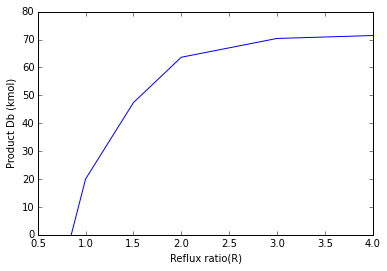The area under the Db vs R curve is given by 96 kmol

Heat to be supplied to provide the reflux,Qr is approximately 384.0 MJ

Heat to be supplied to provide the reflux per kmol of product is then 5.32 MJ

Total heat = 9.32 MJ/kmol product


## Page 596 Example 11.13¶

In :
from numpy import nditer,exp
%matplotlib inline
from matplotlib.pyplot import plot,title,xlabel,ylabel,show

xs=[0.55,0.5,0.425,0.31,0.225,0.105]
xd=[0.78,0.775,0.77,0.76,0.75,0.74]
differ=[]
for m,n in nditer([xd,xs]):
differ.append(m-n)
reci=[]
for i in range(0,6):
reci.append(1/(xd[(i)]-xs[(i)]))

m=[xs, xd, differ, reci]
print"\n xs      xd   (xd-xs)   1/(xd-xs)\n"
for a,b,c,d in nditer([xs,xd,differ,reci]):
print a,'\t',b,'\t',c,'\t%0.3f'%d,
print

plot(xs,reci)
title('Graphical integration')
xlabel('xs')
ylabel('1/xd-xs)')
show()
#Area under the curve is calculated and is found to be 1.1

#logdiv = S1/S2 = area under the curve
logdiv=1.1
S1=100#           #Assume
div=exp(logdiv)
S2=S1/div
Db=S1-S2#        #Product obtained
amt=xs*S1-(xs*S2)
avg=amt/Db

print"\nAverage composition is %.2f kmol\n"%(avg)

L=4000#         #latent heat
R=2.1

h=R*L
print"Heat required to produce reflux per kmol : %d kJ\n"%(h)


 xs      xd   (xd-xs)   1/(xd-xs)

0.55 	0.78 	0.23 	4.348
0.5 	0.775 	0.275 	3.636
0.425 	0.77 	0.345 	2.899
0.31 	0.76 	0.45 	2.222
0.225 	0.75 	0.525 	1.905
0.105 	0.74 	0.635 	1.575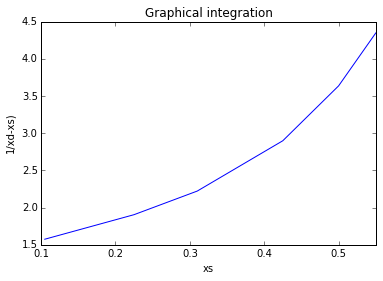Average composition is 0.77 kmol

Heat required to produce reflux per kmol : 8400 kJ



## Page 601 Example 11.14¶

In :
from scipy.optimize import fsolve
from sympy import symbols,solve

xdo = 0.98#               #per cent of ortho top product
xwo = 0.125#              #per cent of ortho bottom product

def product(x):
f=[]
f.append(100 - x - x)#      #x(1) is D and x(2) is W
f.append(60 - x*xdo - x*xwo)
return f
x = [0,0]
y = fsolve(product,x)
print"\n D = %.2f kmol & W = %.2f kmol"%(y,y)

print"\n Let us assume that the distillate contains 0.6 mole per cent meta and 1.4 mole per cent para"
print"\n Component            Feed                       Distillate                 Bottoms         "
print"\n               (kmol)   (mole per cent)     (kmol)  (mole per cent)   (kmol)    (mole per cent)  "
print"\n Ortho        %.3f      %.2f                 %.2f        %.2f            %.2f          %.2f         "%(60,60,y*0.98,98,y*0.125,12.5)
print"\n Meta          %.3f      %.2f                  %.2f        %0.2f            %.2f          %.2f         "%(4,4,y*0.006,0.6,y*0.083,8.3)
print"\n Para         %.3f      %.2f                 %.2f        %.2f            %.2f          %.2f        "%(36,36,y*0.014,1.4,y*0.792,79.2)

ao = 1.7#             #relative volatility of ortho relative to para
am = 1.16#            #relative volatility of meta relative to para
ap =1#                #relative volatility of para w.r.t. to itself
xso = 0.125
xsm = 0.083
xsp = 0.792
xwo = 0.125
xwp = 0.083
xwm = 0.792
yso = ao*xso/(ao*xso+ap*xsp+am*xsm)
ysm = am*xsm/(ao*xso+ap*xsp+am*xsm)
ysp = ap*xsp/(ao*xso+ap*xsp+am*xsm)
#Equations of operating lines
#Above the feed point
Ln = 5*y#          #Liquid downflow
Vn = 6*y#          #Vapour up
#Assuming the feed is liquid at its boiling point
F = 100#           #feed
Lm = Ln+F#         #liquid downflow
Vm = Lm-y#      #Vapour up
x1o = symbols('x1o')
x11 = solve(yso - (Lm/Vm)*x1o + (y/Vm)*xwo)
x1p = symbols('x1p')
x12 = solve(ysp - (Lm/Vm)*x1p + (y/Vm)*xwp)
x1m = symbols('x1m')
x13 = solve(ysm - (Lm/Vm)*x1m + (y/Vm)*xwm)
x1 = [x11, x13, x12]
ax1 = [ao*x11, am*x13, ap*x12]
y1 = [ax1/(ax1+ax1+ax1), ax1/(ax1+ax1+ax1), ax1/(ax1+ax1+ax1)]
x2o = symbols('x2o')
x21 = solve(y1 - (Lm/Vm)*x2o + (y/Vm)*xwo)
x2p = symbols('x2p')
x22 = solve(y1 - (Lm/Vm)*x2p + (y/Vm)*xwp)
x2m = symbols('x2m')
x23 = solve(y1 - (Lm/Vm)*x2m + (y/Vm)*xwm)
x2 = [x21, x23, x22]
print"\n          plate compositions below the feed plate:"
print"\n Component           xs            axs           ys          x1              ax1          y1           x2"
print"\n     o              %.3f           %.3f          %.3f       %.3f         %.3f        %.3f        %.3f"%(xso,ao*xso,yso,x1,ax1,y1,x2)
print"\n     m              %.3f           %.3f          %.3f       %.3f         %.3f        %.3f        %.3f"%(xsm,am*xsm,ysm,x1,ax1,y1,x2)
print"\n     p              %.3f           %.3f          %.3f       %.3f         %.3f        %.3f        %.3f"%(xsp,ap*xsp,ysp,x1,ax1,y1,x2)
print"\n                    %.3f           %.3f          %.3f       %.3f         %.3f        %.3f        %.3f"%(xso+xsm+xsp,ao*xso+am*xsm+ap*xsp,yso+ysm+ysp,x1+x1+x1,ax1+ax1+ax1,y1+y1+y1,x2+x2+x2)

ax2 = [ao*x2, am*x2, ap*x2]
y2 = [ax2/(ax2+ax2+ax2) ,ax2/(ax2+ax2+ax2), ax2/(ax2+ax2+ax2)]
x3o = symbols('x3o')
x31 = solve(yso - (Lm/Vm)*x3o + (y/Vm)*xwo)
x3p = symbols('x3p')
x32 = solve(ysp - (Lm/Vm)*x3p + (y/Vm)*xwp)
x3m = symbols('x3m')
x33 = solve(ysm - (Lm/Vm)*x3m + (y/Vm)*xwm)
x3 = [x31, x33, x32]

ax3 = [ao*x3, am*x3, ap*x3]
y3 = [ax3/(ax3+ax3+ax3), ax3/(ax3+ax3+ax3), ax3/(ax3+ax3+ax3)]
x4o = symbols('x4o')
x41 = solve(yso - (Lm/Vm)*x4o + (y/Vm)*xwo)
x4p = symbols('x4p')
x42 = solve(ysp - (Lm/Vm)*x4p + (y/Vm)*xwp)
x4m = symbols('x4m')
x43 = solve(ysm - (Lm/Vm)*x4m + (y/Vm)*xwm)
x4 = [x41, x43, x42]

ax4 = [ao*x4, am*x4, ap*x4]
y4 = [ax4/(ax4+ax4+ax4), ax4/(ax4+ax4+ax4), ax4/(ax4+ax4+ax4)]
x5o = symbols('x5o')
x51 = solve(yso - (Lm/Vm)*x5o + (y/Vm)*xwo)
x5p = symbols('x5p')
x52 = solve(ysp - (Lm/Vm)*x5p + (y/Vm)*xwp)
x5m = symbols('x5m')
x53 = solve(ysm - (Lm/Vm)*x5m + (y/Vm)*xwm)
x5 = [x51 ,x53, x52]

ax5 = [ao*x5 ,am*x5 ,ap*x5]
y5 = [ax5/(ax5+ax5+ax5), ax5/(ax5+ax5+ax5), ax5/(ax5+ax5+ax5)]
x6o = symbols('x6o')
x61 = solve(yso - (Lm/Vm)*x6o + (y/Vm)*xwo)
x6p = symbols('x6p')
x62 = solve(ysp - (Lm/Vm)*x6p + (y/Vm)*xwp)
x6m = symbols('x6m')
x63 = solve(ysm - (Lm/Vm)*x6m + (y/Vm)*xwm)
x6 = [x61, x63, x62]

ax6 = [ao*x6, am*x6 ,ap*x6]
y6 = [ax6/(ax6+ax6+ax6) ,ax6/(ax6+ax6+ax6) ,ax6/(ax6+ax6+ax6)]
x7o = symbols('x7o')
x71 = solve(yso - (Lm/Vm)*x7o + (y/Vm)*xwo)
x7p = symbols('x7p')
x72 = solve(ysp - (Lm/Vm)*x7p + (y/Vm)*xwp)
x7m = symbols('x7m')
x73 = solve(ysm - (Lm/Vm)*x7m + (y/Vm)*xwm)
x7 = [x71, x73, x72]
print"\n Component       ax2            y2               x3              ax3              y3              x4            ax4"
print"\n     o           %.3f           %.3f          %.3f       %.3f         %.3f        %.3f        %.3f"%(ax2,y2,x3,ax3,y3,x4,ax4)
print"\n     m           %.3f           %.3f          %.3f       %.3f         %.3f        %.3f        %.3f"%(xsm,am*xsm,ysm,x1,ax1,y1,x2)
print"\n     p           %.3f           %.3f          %.3f       %.3f         %.3f        %.3f        %.3f"%(xsp,ap*xsp,ysp,x1,ax1,y1,x2)

print"\n Component      y4                x5          ax5          y5          x6          ax6            y6"
print"\n     o           %.3f           %.3f          %.3f       %.3f         %.3f        %.3f        %.3f"%(y4,x5,ax5,y5,x6,ax6,y6)
print"\n     m           %.3f           %.3f          %.3f       %.3f         %.3f        %.3f        %.3f"%(y4,x5,ax5,y5,x6,ax6,y6)
print"\n     p           %.3f           %.3f          %.3f       %.3f         %.3f        %.3f        %.3f"%(y4,x5,ax5,y5,x6,ax6,y6)

ax7 = [ao*x7 ,am*x7 ,ap*x7]
y7 = [ax7/(ax7+ax7+ax7), ax7/(ax7+ax7+ax7) ,ax7/(ax7+ax7+ax7)]
x8o = symbols('x8o')
x81 = solve(yso - (Ln/Vn)*x8o + (y/Vn)*xwo)
x8p = symbols('x8p')
x82 = solve(ysp - (Ln/Vn)*x8p + (y/Vn)*xwp)
x8m = symbols('x8m')
x83 = solve(ysm - (Ln/Vn)*x8m + (y/Vn)*xwm)
x8 = [x81, x83, x82]

ax8 = [ao*x8 ,am*x8 ,ap*x8]
y8 = [ax8/(ax8+ax8+ax8), ax8/(ax8+ax8+ax8) ,ax8/(ax8+ax8+ax8)]
x9o = symbols('x9o')
x91 = solve(yso - (Ln/Vn)*x9o + (y/Vn)*xwo)
x9p = symbols('x9p')
x92 = solve(ysp - (Ln/Vn)*x9p + (y/Vn)*xwp)
x9m = symbols('x9m')
x93 = solve(ysm - (Ln/Vn)*x9m + (y/Vn)*xwm)
x9 = [x91, x93, x92]

print"\n Component      x7                ax7          y7        x8           ax8         y8            x9"
print"\n     o           %.3f           %.3f          %.3f       %.3f         %.3f        %.3f        %.3f"%(x7,ax7,y7,x8,ax8,y8,x9)
print"\n     m           %.3f           %.3f          %.3f       %.3f         %.3f        %.3f        %.3f"%(x7,ax7,y7,x8,ax8,y8,x9)
print"\n     p           %.3f           %.3f          %.3f       %.3f         %.3f        %.3f        %.3f"%(x7,ax7,y7,x8,ax8,y8,x9)

print"\n Component         x7            ax7           y7           x8          ax8           y8         x9"
print"\n     o             %.3f           %.3f          %.3f       %.3f         %.3f        %.3f        %.3f"%(y4,x5,ax5,y5,x6,ax6,y6)
print"\n     m             %.3f           %.3f          %.3f       %.3f         %.3f        %.3f        %.3f"%(y4,x5,ax5,y5,x6,ax6,y6)
print"\n     p             %.3f           %.3f          %.3f       %.3f         %.3f        %.3f        %.3f"%(y4,x5,ax5,y5,x6,ax6,y6)

 D = 55.56 kmol & W = 44.44 kmol

Let us assume that the distillate contains 0.6 mole per cent meta and 1.4 mole per cent para

Component            Feed                       Distillate                 Bottoms

(kmol)   (mole per cent)     (kmol)  (mole per cent)   (kmol)    (mole per cent)

Ortho        60.000      60.00                 54.44        98.00            5.56          12.50

Meta          4.000      4.00                  0.33        0.60            3.69          8.30

Para         36.000      36.00                 0.78        1.40            35.20          79.20

plate compositions below the feed plate:

Component           xs            axs           ys          x1              ax1          y1           x2

o              0.125           0.212          0.193       0.185         0.315        0.272        0.255

m              0.083           0.096          0.087       0.170         0.198        0.171        0.244

p              0.792           0.792          0.719       0.645         0.645        0.557        0.501

1.000           1.101          1.000       1.000         1.157        1.000        1.000

Component       ax2            y2               x3              ax3              y3              x4            ax4

o           0.433           0.356          0.185       0.315         0.272        0.185        0.315

m           0.083           0.096          0.087       0.170         0.198        0.171        0.244

p           0.792           0.792          0.719       0.645         0.645        0.557        0.501

Component      y4                x5          ax5          y5          x6          ax6            y6

o           0.272           0.185          0.315       0.272         0.185        0.315        0.272

m           0.171           0.170          0.198       0.171         0.170        0.198        0.171

p           0.557           0.645          0.645       0.557         0.645        0.645        0.557

Component      x7                ax7          y7        x8           ax8         y8            x9

o           0.185           0.315          0.272       0.252         0.428        0.272        0.252

m           0.170           0.198          0.171       0.232         0.269        0.171        0.232

p           0.645           0.645          0.557       0.877         0.877        0.557        0.877

Component         x7            ax7           y7           x8          ax8           y8         x9

o             0.272           0.185          0.315       0.272         0.185        0.315        0.272

m             0.171           0.170          0.198       0.171         0.170        0.198        0.171

p             0.557           0.645          0.645       0.557         0.645        0.645        0.557


## Page 608 Example 11.15¶

In :
from sympy import symbols,solve

print"\n Dew point calculation"
xd1 = 0.975#             #n-C4 light distillate
xd2 = 0.025#            #C5 heavy key distillate
Td = 344#               #temperature in kelvins
K1 = 1.05#             #Eqillibrium constant calculation for n-C4 at 344 K
K2 = 0.41#             #Equillibrium constant calculation for C5 at 344K
#By a dew point calculation
#sum(xd)=sum(xd/K)
print"\n Component      xd               Td = 344 K"
print"\n                                 K       xd/K "
print"\n n-C4           %.3f            %.2f      %.3f"%(xd1,K1,xd1/K1)
print"\n C5             %.3f            %.2f      %.3f"%(xd2,K2,xd2/K2)
print"\n                %.1f                      %.3f"%(xd1+xd2,(xd1/K1)+(xd2/K2))

K11 = 1.04
K21 = 0.405
#Calculation for xd at 343 K
x = symbols('x')
x1 = solve(x/K11 + (1-x)/K21)
print"\n   Td = 343 K"
print"\n    K     xd/K"
print"\n   %.3f   %.3f"%(K11,x1/K11)
print"\n   %.3f   %.3f"%(K21,(1-x1)/K21)
print"\n   %.3f"%(x1/K11+(1-x1)/K21)
print"\n At 343 K       K1 = 1.04      K2 = 0.405   from fig.11.39"

print"\n\n\n Estimation of still temperature Ts"
#sum(xw) = sum(K*xw)
K31 = 3.05#            #equillibrium const at 419 K
K32 = 1.6#             #equillibrium const at 419 K
K33 = 0.87#            #equillibrium const at 419 K
K34 = 0.49#            #equillibrium const at 419 K
xw1 = 0.017#           #mass fraction of n-C4
xw2 = 0.367#           #mass fraction of C5
xw3 = 0.283#           #mass fraction of C6
xw4 = 0.333#           #mass fraction of C7

print"\n At Ts = 416 K equillibrium constants are from fig.11.39"
print"\n n-C4           C5        C6          C7"
print"\n  %.2f          %.2f      %.2f       %.2f"%(K31,K32,K33,K34)
print"\n\n   at Ts = 416 K"
print"\n n-C4           C5        C6          C7"
print"\n  %.3f          %.3f       %.3f      %.3f"%(xw1*K31,xw2*K32,xw3*K33,xw4*K34)
print"\n Sum of Kxw = %d"%(xw1*K31+xw2*K32+xw3*K33+xw4*K34)
print"\n Hence the still temperature Ts = 416 K"
print"\n\n\n Calculation of feed condition"
print"\n Component     xf         Tb = 377K         Tb = 376 K"
print"\n                         K       Kxf        K     Kxf "
xf1 = 0.40
xf2 = 0.23
xf3 = 0.17
xf4 = 0.20
Kb1 = 1.80#             #equillibrium constants at 377 K for n-C4
Kb2 = 0.81#             #equillibrium constants at 377 K for C5
Kb3 = 0.39#             #equillibrium constants at 377 K for C6
Kb4 = 0.19#             #equillibrium constants at 377 K for C7
Kb11 = 1.78#            #equillibrium constants at 377 K for n-C4
Kb21 = 0.79#            #equillibrium constants at 377 K for C5
Kb31 = 0.38#            #equillibrium constants at 377 K for C6
Kb41 = 0.185#           #equillibrium constants at 377 K for C7
print"\n n-C4         %.2f     %.2f     %.3f      %.2f   %.3f"%(xf1,Kb1,xf1*Kb1,Kb11,xf1*Kb11)
print"\n C5           %.2f     %.2f     %.3f      %.2f   %.3f"%(xf2,Kb2,xf2*Kb2,Kb21,xf2*Kb21)
print"\n C6           %.2f     %.2f     %.3f      %.2f   %.3f"%(xf3,Kb3,xf3*Kb3,Kb31,xf3*Kb31)
print"\n C7           %.2f     %.2f     %.3f      %.2f   %.3f"%(xf4,Kb4,xf4*Kb4,Kb41,xf4*Kb41)
print"\n                                %.3f             %.3f"%(xf1*Kb1+xf2*Kb2+xf3*Kb3+xf4*Kb4,xf1*Kb11+xf2*Kb21+xf3*Kb31+xf4*Kb41)

#Calculation of pinch temperatures
print"\n\n\n The upper pinch temperature,Tn = %d K"%(343+0.33*(416-343))
print"\n The lower pinch temperature,Tm = %d K"%(343+0.67*(416-343))

#Calculation of approximate minimum reflux ratio.
print"\n\n\n"
print"\n Component      Tn = 367 K      Tm = 391 K    xfh   axfh"
print"\n                   a                a                   "
print"\n n-C4              %.2f             %.2f                "%(2.38,2.00)
print"\n C5                %.2f             %.2f                "%(1.00,1.00)
print"\n C6                %.3f             %.3f      %.2f    %.3f"%(0.455,0.464,0.17,0.077)
print"\n C7                %.3f             %.3f      %.2f    %.3f"%(0.220,0.254,0.20,0.044)
print"\n                                                      %.3f"%(0.077+0.044)
rf = xf1/xf2
print"\n rf = %.3f"%(rf)
xn4 = rf/((1+rf)*(1+0.121))
print"\n xn4 = %.3f"%(xn4)
xn5 = xn4/rf
print"\n xn5 = %.3f"%(xn5)
Rm = (1/(2.38-1))*(0.975/0.563)-2.38*(0.025/0.325)
print"\n Rm = %.2f"%(Rm)

#The streams in the column
D = 40
Ln = D*Rm
Vn = Ln+D
F = 100
Lm = Ln + F
W = 60
Vm = Lm - W
Ratio = Lm/W
print"\n Ln = %.1f kmol"%(44.8)
print"\n Vn = %.1f kmol"%(84.8)
print"\n Lm = %.1f kmol"%(144.8)
print"\n Vn = %.1f kmol"%(84.4)
print"\n Lm/W = %.2f"%(Ratio)
#Check on minimum reflux ratio
#xn = xd/(a-1)Rm
xn = xd1/((2.38-1)*Rm)
print"\n For n-C4......xn = %.3f"%(xn)
xn1 = 1-xn
print"\n For n-C5...xn = %.3f"%(xn1)
print"\n Temperature check for upper pinch gives sum of K*xn = "
sumKxn = 1.62*xn +0.68*xn1
print"%.3f"%(sumKxn)

 Dew point calculation

Component      xd               Td = 344 K

K       xd/K

n-C4           0.975            1.05      0.929

C5             0.025            0.41      0.061

1.0                      0.990

Td = 343 K

K     xd/K

1.040   1.575

0.405   -1.575

-0.000

At 343 K       K1 = 1.04      K2 = 0.405   from fig.11.39

Estimation of still temperature Ts

At Ts = 416 K equillibrium constants are from fig.11.39

n-C4           C5        C6          C7

3.05          1.60      0.87       0.49

at Ts = 416 K

n-C4           C5        C6          C7

0.052          0.587       0.246      0.163

Sum of Kxw = 1

Hence the still temperature Ts = 416 K

Calculation of feed condition

Component     xf         Tb = 377K         Tb = 376 K

K       Kxf        K     Kxf

n-C4         0.40     1.80     0.720      1.78   0.712

C5           0.23     0.81     0.186      0.79   0.182

C6           0.17     0.39     0.066      0.38   0.065

C7           0.20     0.19     0.038      0.18   0.037

1.011             0.995

The upper pinch temperature,Tn = 367 K

The lower pinch temperature,Tm = 391 K

Component      Tn = 367 K      Tm = 391 K    xfh   axfh

a                a

n-C4              2.38             2.00

C5                1.00             1.00

C6                0.455             0.464      0.17    0.077

C7                0.220             0.254      0.20    0.044

0.121

rf = 1.739

xn4 = 0.566

xn5 = 0.326

Rm = 1.07

Ln = 44.8 kmol

Vn = 84.8 kmol

Lm = 144.8 kmol

Vn = 84.4 kmol

Lm/W = 2.38

For n-C4......xn = 0.659

For n-C5...xn = 0.341

Temperature check for upper pinch gives sum of K*xn =
1.300


## Page 612 Example 11.16¶

In :
#Mole fractions
xf=[0.40, 0.35 ,0.25]
xd=[0.534, 0.453, 0.013]
xw=[0 ,0.04 ,0.96]

#Amount of feed, product, bottom in kmol
F=[40, 35, 25]
D=[40, 34, 1]
W=[0 ,1, 24]

#roots of equation
theta=[1.15, 1.17]

#relative volatility
alpha=[2.7 ,2.22, 1]

#Underwoods 1st equation for q=1
sums=[0 ,0]
for i in range(0,1):
for j in range(0,2):
sums[i]=sums[i]+(alpha[j]*xf[(j)]/(alpha[(j)]-theta[(i)]))

print"\nFrom Underwoods 1st eq\n"
print"The value of 1-q at theta = 1.15 and 1.17 are"
print sums

#Underwoods 2nd equation for minimum reflux ratio
sum2=0
for l in range(0,2):
sum2=sum2+(alpha[(l)]*xd[(l)]/(alpha[(l)]-theta))

Rm=sum2-1
print"\nMinimum Reflux ratio is %.3f\n"%(Rm)

From Underwoods 1st eq

The value of 1-q at theta = 1.15 and 1.17 are
[1.4229424178474521, 0]

Minimum Reflux ratio is 0.900



## Page 613 Example 11.17¶

In :
from math import log
#From previous question data
xA_d=0.453
xB_d=0.013

xA_s=0.04
xB_s=0.96

alpha_av=2.22

#By Fenske Equation for no. of plates

n=((log(xA_d*xB_s/(xA_s*xB_d)))/log(alpha_av))-1

print"\nMinimum no. of plates are %.3f   or %d\n"%(n,n)

Minimum no. of plates are 7.438   or 7



## Page 614 Example 11.18¶

In :
from sympy import symbols,solve
R=[1 ,2 ,5, 10]
Rm=0.83

nm=8.5-1

#X are points on X-axis of graph
X=[]
for i in range(0,3):
X.append((R[(i)]-Rm)/(R[(i)]+1))

#Values at Y-axis for corresponding values of X-axis in graph are given by
Y=[0.55, 0.32 ,0.15, 0.08]

#where Y=(n+1)-(nm+1)/(n+2)

N=[]
for i in range(0,3):
n=symbols('x')
N.append(solve(((n+1)-(nm+1))-(Y[(i)]*(n+2))))

print"\nThe values of R and n are\n"
for i in range(0,3):
print"\n\t%d \t %.2f"%(R[i],N[i])

print"\n\nThe change in R and n can be seen as above\n"

The values of R and n are

1 	 19.11

2 	 11.97

5 	 9.18

The change in R and n can be seen as above



## Page 615 Example 11.19¶

In :
%matplotlib inline
from matplotlib.pyplot import plot,title,xlabel,ylabel,show

#Data from fig. 11.42
a = [0, 0.02, 0.04, 0.06, 0.08, 0.1 ,0.2 ,0.4 ,0.6 ,0.8 ,1.0]
b = [0.75, 0.62, 0.60, 0.57, 0.55, 0.52, 0.45, 0.30, 0.18, 0.09, 0]
#a = (R-Rm)/(R+1)
#b = [(n+1)-(nm+1)]/(n+2)
R = [0.92, 1.08, 1.25 ,1.75 ,2.5 ,3.5, 5.0 ,7.0 ,9.0]
n = [28.6 ,22.8 ,16.9 ,13.5 ,11.7 ,10.5 ,9.8 ,9.2 ,8.95]
plot(n,R)
title("Plot of R vs n")
xlabel("n")
ylabel("R")
show()
print"\n Derivative calculated from the graph"
d = [110.0, 34.9 ,9.8 ,3.8 ,1.7 ,0.6 ,0.4 ,0.2 ,0.05]
i=0
while i <=8:
s = R[(i)]+1 - (n[(i)]+7.72)/d[(i)]
if s <=0.0001:
Ropt = R[(i)]
print"\n Ropt = %.2f"%(Ropt)
break

i=i+1

print"\n R is approximately %.1f percent of the minimum reflux condition"%(1.25/0.866666666*100)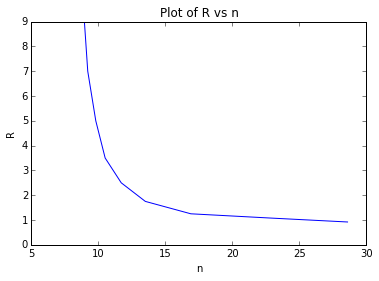Derivative calculated from the graph

Ropt = 1.25

R is approximately 144.2 percent of the minimum reflux condition


## Page 633 Example 11.20¶

In :
from math import log10

#Mole fraction
xf=[0.2, 0.3, 0.2, 0.3]

#Viscosity at mean tower temp. in mNs/m**2
uL=[0.048, 0.112, 0.145, 0.188]

#Viscosity of water in mNs/m**2
uw=1

sums=0
for i in range(0,3):
sums=sums+(xf[(i)]*uL[(i)]/uw)


Efficiency is 0.87